# SAT Math Multiple Choice Question 190: Answer and Explanation

### Test Information

Question: 190

10. Line d has a slope of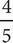and passes through the point (1, 1). Line e is parallel to line d and has a y-intercept 3 times that of line d. Which of the following is the equation of line e ?

• A. 5y - 4x = 3
• B. 5y - x = 4
• C. 10y - 8x = 30
• D. 20y + 25x = 12

A The equation of a line expressed in slope-intercept form is y = mx + b, where m is the slope and b is the y-intercept. One way to find the y-intercept of line d is to plug in the slope and given point and slope for b. The equation y = mx + b becomes 1 =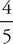(1) + b. Subtractfrom both sides to get b =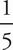. Another approach is to use the slope formula to find b. The y-intercept of a line is where the line crosses the y-axis; at that point x = 0. Therefore, in addition to the point (1, 1), there is another point (0, b) that lies on line d. The equation for finding the slope of the line given two points is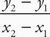. Therefore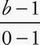=or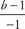=. Cross-multiply to get 5(b - 1) = -4. Distribute the 5 to get 5b - 5 = -4. Solve for b to get 5b = 1, and b =. The y-intercept of line e is 3 times, so the y-intercept of line e is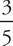. Additionally, parallel lines have slopes that are equal to each other. Therefore, line e will also have a slope equal to. Rewrite the equation in (A) in the slope-intercept form of the equation to get 5y = 4x +3, or y =x +. The slope of this line isand the y intercept is. Therefore, the correct answer is (A).